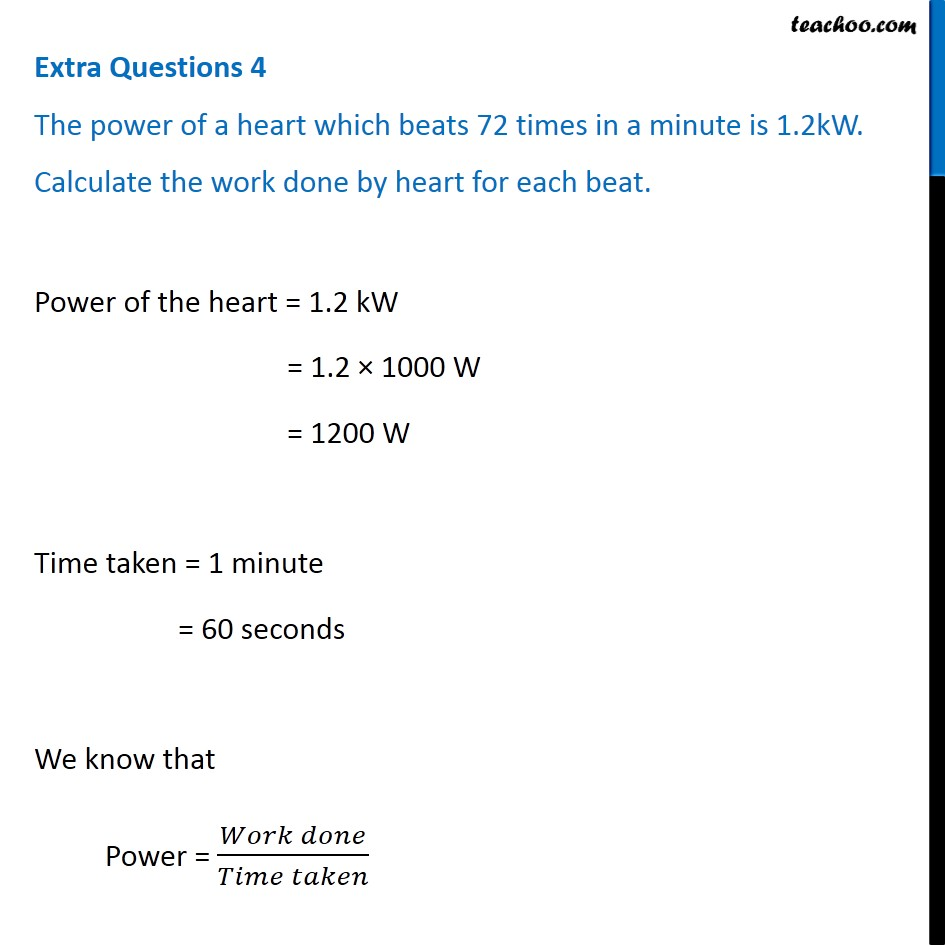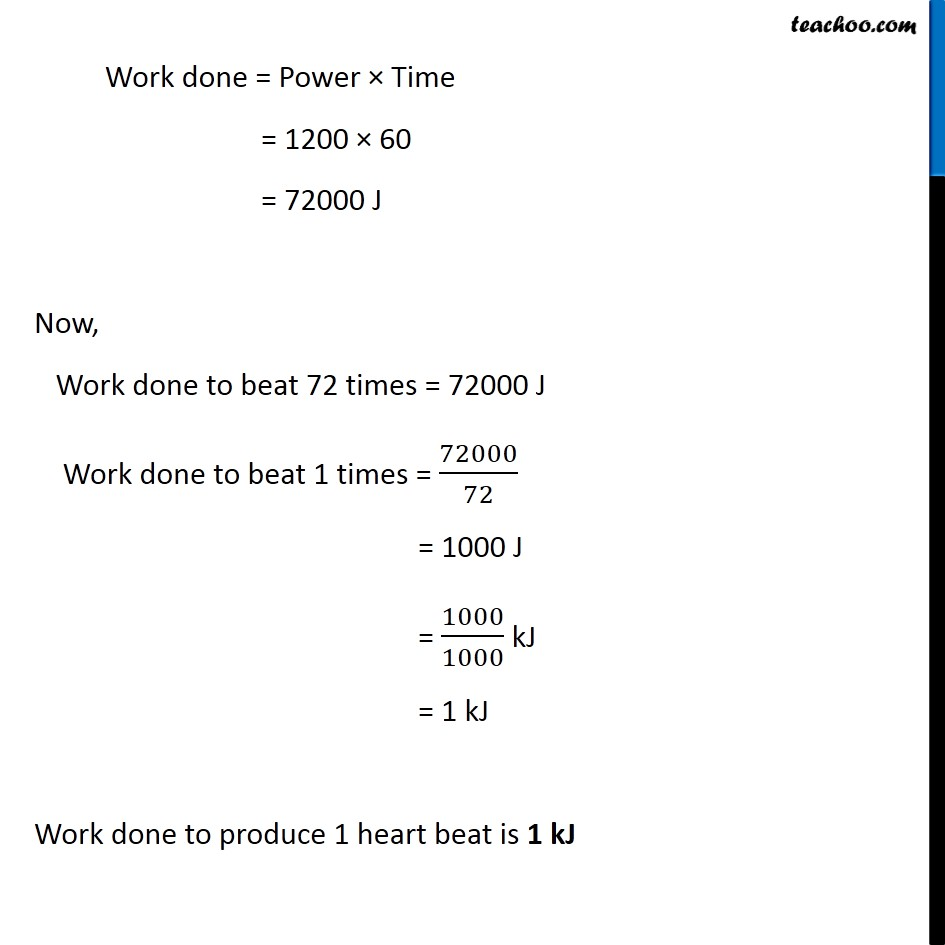Teachoo Questions

Class 9
Chapter 11 Class 9 - Work and Energy

## The power of a heart which beats 72 times in a minute is 1.2kW. Calculate the work done by heart for each beat.Learn in your speed, with individual attention - Teachoo Maths 1-on-1 Class

### Transcript

Extra Questions 4 The power of a heart which beats 72 times in a minute is 1.2kW. Calculate the work done by heart for each beat. Power of the heart = 1.2 kW = 1.2 × 1000 W = 1200 W Time taken = 1 minute = 60 seconds We know that Power = (𝑊𝑜𝑟𝑘 𝑑𝑜𝑛𝑒)/(𝑇𝑖𝑚𝑒 𝑡𝑎𝑘𝑒𝑛) Work done = Power × Time = 1200 × 60 = 72000 J Now, Work done to beat 72 times = 72000 J Work done to beat 1 times = 72000/72 = 1000 J = 1000/1000 kJ = 1 kJ Work done to produce 1 heart beat is 1 kJ